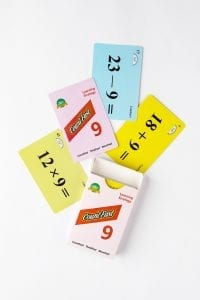# CountFast 9 Home Activities

###OVERVIEW & PURPOSE

Week 5 of the 3rd Grade CountFast program focuses on strategies to add, subtract, and multiply with the digit 9.  Spend 15 minutes each day on one of the activities listed in this module.  Card decks should go home with students each day for additional practice with a parent at home. Each week, a new deck is introduced, and the previous deck is for the student to keep at home for continued practice.

#### EDUCATION STANDARDS

1. NCTM Standard: develop a sense of whole numbers and represent and use them in flexible ways, including relating, composing, and decomposing numbers
2. Math.Content.3.OA.B.5
Apply properties of operations as strategies to multiply and divide.

#### OBJECTIVES

1. Mentally add and subtract with the number nine.
2. Mentally multiply one and two-digit numbers by nine.
DAY 1:Today, your child learned how to quickly add 9 to any number by thinking of the number 9 as being the same thing as 10 – 1.  To add nine quickly, first add 10 to the original number, and then back up one number.  For example, to add 18 + 9, first realize that 18 + 10 is 28, then back up one spot on the number line, which would be 27 for a final answer.  Practice with your child using the yellow cards.  You can use the time record card to keep track of how quickly your child can add all of the yellow cards each round of practice.DAY 2:

Use the same procedure as yesterday.  This time, your child will use the blue cards in the deck to practice subtracting 9 from any number.  Using the idea that 9 is the same thing as 10 – 1, first subtract 10 from the original number, and then go back up one number for the final answer.  For example, solve 23 – 9 by first subtracting 10.  This puts you at 13.  Then move up (in a positive direction) on the number line one spot, which gives the final answer of 24.  Practice with your child using the blue cards.  Record the speed your child completes the blue cards each round of practice.

DAY 3:

Today, we learned a neat trick using our fingers to quickly find the answer to any single-digit number times 9.  Holding both hands up in front of you, think about ‘reading’ the fingers as 1 through 10, going from left to right.  Hold down the finger that 9 is being multiplied by – for example, 9 X 3 – hold down the third finger.  The fingers on the left of the one held down represent the ‘tens’ number of the answer.  The fingers on the right represent the ‘ones’ digit of the answer.  For 9 X 3, there are 2 fingers on the left, and 7 on the right, giving the answer of 27.  Have your child model the technique for you.  Then quickly ask your child various ‘9 times’ facts to see how quickly he/she can get the answer using this method.

DAY 4:

Today your child learned a strategy to multiply two-digit numbers by 9.   The quick way to mentally multiply by 9 is to multiply the original number by 10 (by simply adding a zero to the original number) and then subtract the original number to get the final answer.  For example, 15 X 9 is solved quickly by first multiplying 15 X 10 to get 150, and then subtracting 15 from 150 to get a final answer of 135.  Practice this method with your child using the green cards in the deck.  Record your child’s speed through the cards each round of practice.

DAY 5:

Today we reviewed all of the strategies for the number 9.  Shuffle the deck and show your child one problem at a time. The addition, subtraction, and multiplication problems will be randomly scattered when shuffled – so your child will have to pay careful attention to the type of problem on the card in order to choose the right strategy for solving.  Make it a family game by having multiple members of the family race to answer correctly first!

Stay SharpFree flash card decks to keep kids entertained and sharp!

To help you keep your kids math sharp during this crisis, we're giving you 3 free CountFast flash card decks for just \$6 in shipping.  Just have them take the quick quiz for their grade at the link below and you'll get a discount code to use on any three decks.